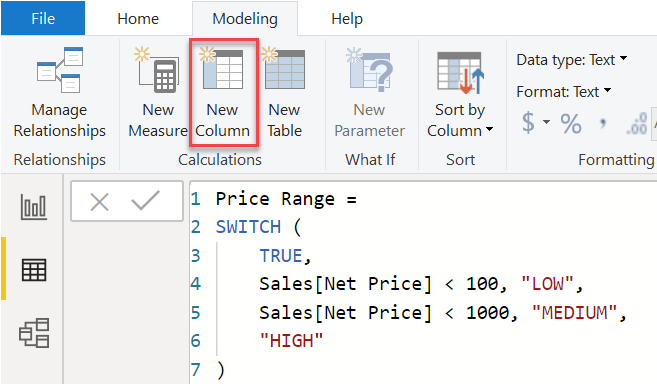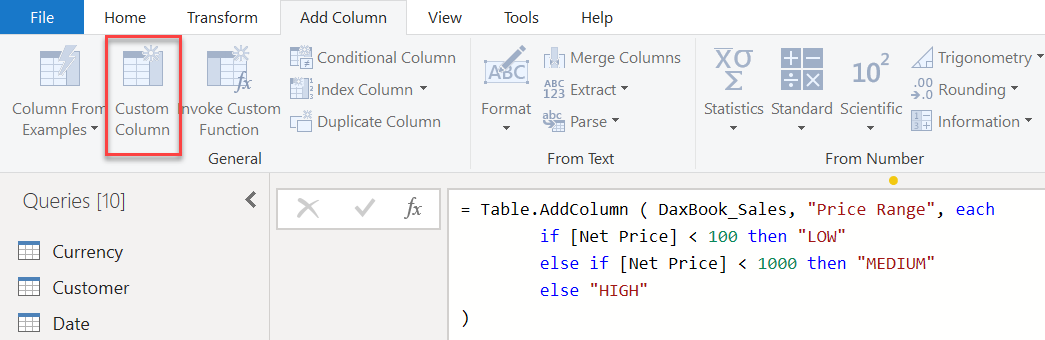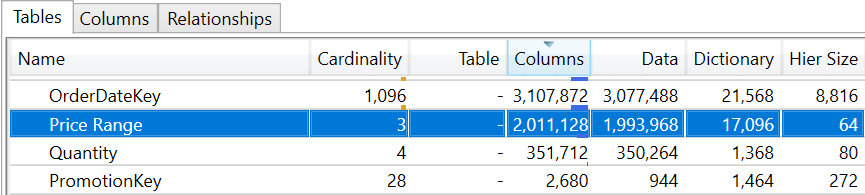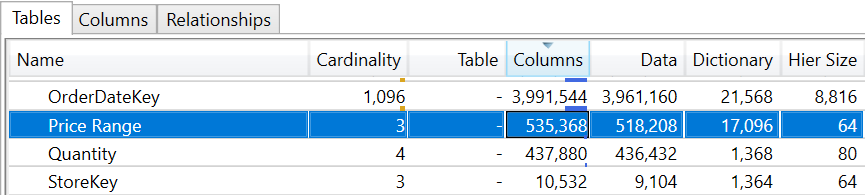When building a data model in Power BI, Analysis Services, or Power Pivot, you might need to extend a table coming from a data source with additional columns, whose content derives from existing data. Several features are available to create such additional columns in the tools mentioned above, and choosing the right feature can sometimes be a challenge. This article describes the main differences between calculated columns based on DAX expressions and computed columns generated by Power Query. As you will see, the choice of a DAX calculated column should be limited to cases where the result is obtained by accessing data in different rows of the same table or in different tables; you should however choose a Power Query computed column when the business logic to implement relies on the values of other columns of the same table.

IMPORTANT: a DAX calculated column in a DirectQuery model is evaluated at query time similarly to what happens with Power Query computed columns. This article only applies to models where you import data in memory.

## Introducing DAX calculated columns

A DAX calculated column computes the result of a DAX expression after the content of the model has been imported in memory. The DAX expression is executed for every row of a table, can use columns of the same table through the row context, and can aggregate data using the filter context that is initially empty. The result of the DAX expression evaluated for every row is stored in memory as other columns of the table; this result can be used in other calculations and to filter and group data.

The following screenshot shows a Price Range calculated column in the Sales table, which only depends on the values of the Sales[Net Price] column.## Introducing Power Query computed columns

A Power Query computed column is an M expression that defines the transformations to apply to a data source before importing a table in the data model. Depending on the expression and on the data source, the M expression of a Power Query computed column can be translated into an equivalent request to the data source – like an expression of a column in a SQL query ; it can also be computed by the Power Query engine, modifying the rows coming from the data source that are used to populate the table in the data model.

The following screenshot shows a Price Range computed column in the query for the Sales table that depends on the value of the Net Price column.## Differences between DAX calculated columns and Power Query computed columns

This is a list of the most important differences between DAX calculated columns and Power Query computed columns considering specific scenarios.

• Adding a column to the model
• A new DAX calculated column does not require a full refresh of the table.
• A new Power Query computed column requires a full refresh of the table.
• Full refresh of the table
• The engine processes DAX calculated columns after the data of all the partitions has been loaded and compressed in memory.
• A Power Query computed column is processed like any other column coming from the data source.
• Incremental refresh and partition refresh
• The engine processes the DAX calculated columns for all the rows of the table, even when only a few rows are added to or refreshed in the table.
• The engine only computes values for the Power Query computed columns of the rows that are processed in any partial refresh.
• Comparing compression
• A DAX calculated column does not participate in the evaluation of the best sort order for the compression. As a result, the compression of the column might be far from ideal, especially for columns with a low number of unique values.
• A Power Query computed column is compressed like any other column of the table.
• Processing time
• The cost of processing a single DAX calculated column corresponds to a sequential evaluation of the DAX expression for each row included in a refresh operation. Multiple DAX calculated columns within the same table are processed sequentially, one after the other, with an order compatible with calculation dependencies.
• The cost for processing a Power Query calculated column mostly depends on the data source when the expression is optimized using query folding. For example, if M expressions are translated into equivalent SQL expressions, performance and parallelism entirely depend on the SQL data source.
• Improving the compression of one column might negatively impact the compression of other columns in the data model.
• Query performance
• A column with better compression is smaller in memory and usually provides better performance levels. This is important in filter, group, and aggregation operations involving the column.
• The compression of a DAX calculated column might be lower than that of a Power Query computed column.
• An improvement in the compression of one column might negatively affect the compression of other columns in the data model.

## Explaining different compression results

A calculated column with a small number of unique values does not benefit from changing the sort order of the table. This actually results in less efficient compression. For example, we created the Price Range column using the two techniques described above in a Sales table with more than 12 million rows. VertiPaq Analyzer shows that the data size of the Price Range calculated column in DAX is 1,993,968 bytes.By creating Price Range as a computed column in Power Query, the data size is only 518,208 bytes.Though you eliminate almost 75% of the size of the Price Range column, this does not necessarily mean that you saved the same number of bytes (1,475,760) from the entire database size. Other columns might have a worse compression. For example, both the Quantity and OrderDateKey columns visible in the two previous figures saw their size increase significantly. In this example the model size increased with the computed column, as you can see from the VertiPaq files available for download. However, the result obtained by changing a single column in a model is almost unpredictable. Nevertheless, if you are considering adding multiple calculated or computed columns, then computed columns are usually a better choice even though the overall result may vary case by case.

## When to use Power Query computed columns

You should use Power Query computed columns whenever the expression only depends on other columns of the same row of the table where you create the new column. This is the case for the Price Range column used in the example of this article.

If the data source is a relational database and you merge tables that can be joined in the data source efficiently, then it is a good idea to denormalize a table using Power Query functions – rather than importing multiple tables into the data model and then creating DAX calculated columns retrieving corresponding data from other tables using RELATED.

You should be careful when deciding whether to use Power Query computed columns, if you need to aggregate rows from other tables. The Power Query computed columns executes the aggregation on the data source, and this might take a long execution time. If this happens, you should consider a DAX calculated column to avoid a long processing times.

## When to use DAX calculated columns

You should use DAX calculated columns whenever you cannot implement the same calculation in a Power Query computed column. The typical case is when aggregating data from other tables in the model. Another case would be the denormalization of tables coming from different data sources, because this would not be optimized using the query folding technique in Power Query.